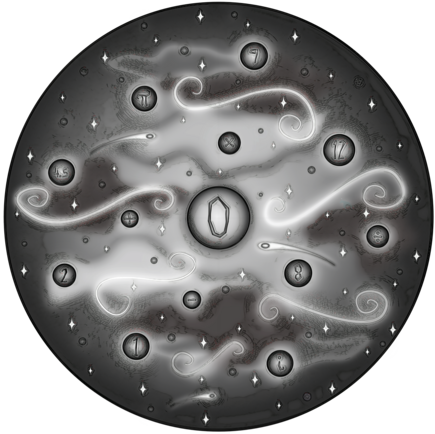The Zero Universe

In the beginning there was Zero,

And Zero was all there was;

Zero never did much,

As nothing never does.

But then one fateful moment

Zero had a thought;

Zero started thinking

Of all that it was not.

Then Zero started visualizing

All that it could be,

And soon it came to realize

It could be anything.

Zero could potentially be

2 plus 8 plus 3,

As long as it was sure to add

Negative 13.

It could be 12 divided by 2

Then multiplied by 9,

If it also negatively added

12 times 4.5.

It could even be i times pi

Then e to the power of that;

As long as 1 was added too,

Zero was still intact.

But these kinds of equations needed

Some chronology;

Calculations required time,

So time came into being.

Now Zero could express itself

With separate entities,

A daunting infinite amount

Of possibilities.

But then an unexpected thing

Soon began to occur;

These new and separate entities

Started to observe.

They first became aware of themselves,

Then they looked out far,

And then they all began to notice

Other equation parts.

9 saw 12 divided by 2,

3 noticed 4.5;

e discovered 8 plus 3,

1 saw i times pi.

They observed each other curiously

And tried to do the math.

What did these random numbers mean?

They craved to understand.

Only Zero knew the answer to

The equation in its head:

It’s always been and will always equal

Zero in the end!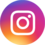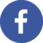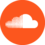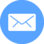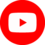• Home